## 1. Introduction

When you type tar -zcf src.tar.gz src, you can package all the files under src into a tar.gz format. Here “tar” is the archive format, which combines multiple files into a single file, and “gz” refers to the gzip compression format, which uses the DEFLATE algorithm to compress. As the most widely used lossless compression algorithm, how does DEFLATE work and what are the principles behind it? In this article, we will discuss this issue.

DEFLATE combines the LZ77 algorithm with Huffman coding, designed by Phil Katz and standardized by RFC1951. This paper is a summary of my research on RFC1951 and zlib, introducing first the LZ77 algorithm, then the role of Huffman encoding in DEFLATE, and finally the Finally, we introduce the format of gzip.

## 2. LZ77 Algorithm

J. Ziv and A. Lempel published a paper in 1977 called A Universal Algorithm for Sequential Data Compression, which proposed a universal algorithm for compressing sequential data. This algorithm became known as the LZ77 algorithm. In fact, the DEFLATE algorithm uses a different version of LZ77 than the original, so here we use the one in DEFLATE.

### 2.1 Basic principles

The LZ77 compression algorithm takes advantage of the fact that there are always a lot of duplicates in the files we use. It tries to find as many duplicates as possible in the file, and then replaces them with a marker that clearly indicates where they occur. As an example, take the first paragraph of the Gettysburg Address:

Four score and seven years ago our fathers brought forth, on this continent, a new nation, conceived in Liberty, and dedicated to the proposition that all men are created equal.

We can find quite a few duplicate fragments. We specify that whenever more than two characters are duplicated, the duplicate token <d,l> is used in its place. <d,l> means that the string at this position is equivalent to a string of length l before the d character. So the above can be expressed as:

Four score and seven years ago <30,4>fathe<16,3>brought forth, on this continent, a new nation,<25,4>ceived in Liberty<36,3><102,3>dedicat<26,3>to<69,3>e proposi<56,4><85,3>at all m<138,3>a<152,3>cre<44,5>equal.

Well, you may have noticed that the result of this “compression” is even longer than the original. Don’t worry, this is just a demonstration, and we’ll see how to solve this problem later.

The simplest way to implement this algorithm is to iterate through the string, looking for duplicates in the preceding order for each character, and then finding the longest duplicate substring. This approach has a time complexity of $\mathrm{O}(n^2)$, which is inefficient, especially when working with large files. To improve efficiency, we use hash table and sliding window optimization.

### 2.2 Using Hash Tables

Sequential lookups are too slow, so we can use a hash table to improve efficiency. We use a hash table to map a string of length three to a list of all occurrences of the string. Compression scans the string from beginning to end. Suppose the next three characters are XYZ , first check if the string XYZ is in the hash table. If not, output the character X as is and move it to the next character. Otherwise, compare the string starting from the current position with all the strings starting from the position where XYZ appears, find the longest match of length N and output the duplicate marker, then move N characters backward. Whether or not a match is found in the hash table, the current position is inserted into the list mapped by XYZ in the hash table.

As an example, consider the string abcabcdabcde , the algorithm runs with the following steps:

1. Scan to the 0th character a, the hash table is empty, output the character a as is, and insert the string abc into the hash table.

 1 2 3 4  hash table: {} abcabcdabcde ^ output: a 
2. Scanning to the 3rd character a , the hash table indicates that abc occurs at 0. The longest match is abc , so the output is <3,3> . The current position is also inserted into the list of abc mappings.

 1 2 3 4  hash table: {"abc":,"bca":,"cab":} abcabcdabcde ^ output: abc<3,3> 
3. Scanning to the 7th character a, the hash table indicates that abc appears at 0, 3. Matching the current position in turn, 0 can only match abc , while 3 can match abcd , the longest match is abcd , so the output is <4,4> . The current position is also inserted into the list of abc mappings.

 1 2 3 4  hash table: {"abc":[0,3],"bca":,"cab":,"bcd":,"cda":,"dab":} abcabcdabcde ^ output: abc<3,3>d<4,4> 

BTW, the implementation of this hash table in zlib is very clever and the details of its implementation are beyond the scope of this article. Anyone interested in the C language should check out this implementation.

### 2.3 Sliding windows

Even with a hash table, overly long files can still cause efficiency problems. For this reason we use a sliding window, where duplicates are found only in the window. The length of the window is fixed, usually 32KB. In the zlib implementation, a buffer twice the size of the window is used, as shown below:

 1 2  |-------search-------+------lookahead-----| ^ 

Initially, the data to be compressed is filled into the buffer, and the pointer is located at the beginning of the buffer; the pointer then scans backwards to compress the data. We call the part scanned by the pointer the search area and the part not scanned the lookahead area. For each scanned character, the algorithm only looks for a match in the search area. When the lookahead area is not long enough (less than a fixed value), the algorithm moves the window to continue scanning for more data. This is done by copying the data from the second half of the buffer to the first half and moving the pointer, then reading in the new data in the second half of the buffer, and then updating the hash table as the location of the data changes.

### 2.4 lazy matching

Sometimes this strategy does not give the best compression result. As an example, consider the string abcbcdabcda, which looks like this when the 6th character is scanned:

 1 2 3 4  hash table: {"abc":,"bcb":,"cbc":,"bcd":,"cda":,"dab":} abcbcdabcda ^ output: abcbcd<6,3> 

The hash table indicates that abc appears at 0, the longest match is abc, so <6,3> is output; the next da has no duplicates and is output as is. The result of the compression is abcbcd<6,3>da . However, a longer match bcda can be found in the preceding text at the 7th character position, and a better compression result would be abcbcda<4,4> .

To solve this problem, DEFLATE uses a strategy called inert matching to optimize. Whenever the algorithm finds a match of length N, it tries to find a longer match on the next character. Only if no longer match is found, a duplicate token is output for the current match; otherwise, the current match is discarded and a longer match is used. Note that inert matches can be triggered consecutively; if a longer match is found in one inert match, inert matching will continue until a longer match cannot be found on the next character.

Inert matching can improve compression, but it can slow down compression. Runtime parameters control inert matching, which is only triggered if the current match is not long enough, i.e., if it is less than a configured value. This value can be adjusted depending on whether compression rate or speed is preferred, or inertia matching can be turned off.

## 3. Huffman encoding

It would be silly if we actually used a format like <d,l> to represent repetitive content. First, it takes up so much space that the Gettysburg Address in section 2.1, which is “compressed” in this way, is longer than even the original text. Secondly, this format turns the brackets into special characters, and you have to escape them somehow. So how do you represent the distance and length of the repeated parts? DEFLATE’s approach is to break the old order and create a new one - to re-encode the data using Huffman encoding.

Huffman encoding was introduced by American computer scientist David A. Huffman in 1952, and is a prefix encoding constructed from the frequency of characters. Unlike our usual fixed-bit encoding (8 bits per character), Huffman encoding may have different bits for each character, but can clearly distinguish each character without any ambiguity.

### 3.1 Prefix encoding

How is it that each character has a different number of bits but can be clearly distinguished from the others? This is where prefix encoding comes in. To illustrate what prefix coding is, imagine that we are talking on the phone. The length of a phone number varies: there are three-digit numbers, such as 110, 119; five-digit numbers, such as 10086, 10010; 7- or 8-digit landlines, 11-digit mobile numbers, etc. However, the numbers are always correctly identified when dialed in sequence (no need to press the call button for landline off-hook dialing). This is because no phone number starts with 110 except for the police 110, and no landline number can be the prefix of a cell phone number. A phone number is a prefix code. So, although each character in Huffman encoding has a different number of bits, the shorter encoding will not be the prefix of the longer encoding. This ensures that there is no ambiguity in the Huffman encoding.

We use Huffman trees to construct Huffman encodings. As mentioned above, Huffman encodings have both long and short lengths, so it makes sense to keep the more frequently used characters short and the more frequently used characters long. Suppose we want to encode the characters a, b, c, d, and e, and their frequencies are shown in the following table:

character times
a 1
b 4
c 4
d 3
e 1

The construction is very simple. We consider the frequency of characters as priority and put them in a minimum priority queue: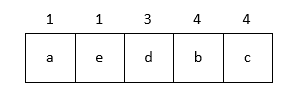Then the first binary tree is constructed by popping the two lowest priority characters as children; the priority of the parent node is considered as the sum of the two byte priorities, and then the parent node is inserted into the queue: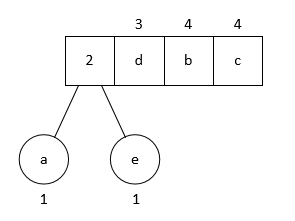Repeating this operation, we end up with a binary tree. This is the Huffman tree.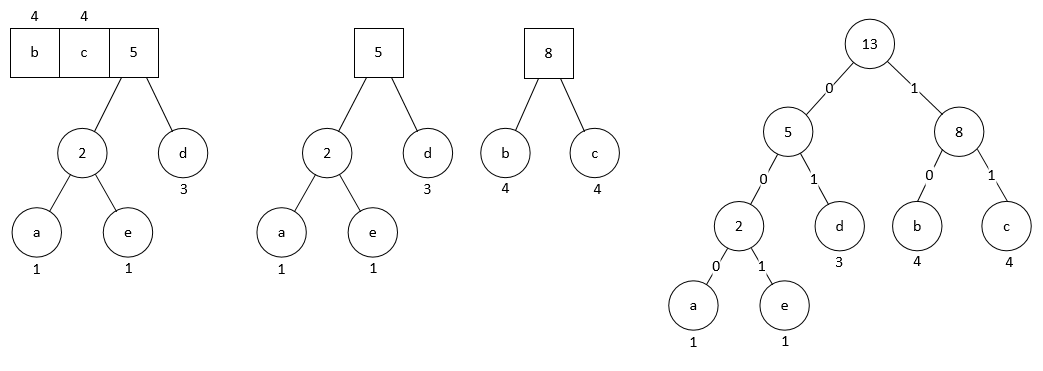If we encode the left branch of the tree as 0 and the right branch as 1, then by traversing from the root node down to the leaf nodes, we can obtain the Huffman encoding of the corresponding characters. So we get the Huffman encoding table for the above example as:

character code
a 000
b 10
c 11
d 01
e 001

Suppose we have the string badbec, and after using Huffman encoding we get 10000011000111. Decoding is also simple, read each bit in turn, use Huffman tree, start from the root node 0 to the left and 1 to the right, and output the corresponding character when it reaches the leaf node, then go back to the root node to continue scanning.

### 3.2 Encoding distance and length

A byte has 8 bits, so we need 256 Huffman encodings from 0 to 255. The frequency of each character in the file is counted, and a Huffman tree is constructed to calculate these 256 codes. How do we represent the distance and length in the repetition mark with Huffman codes? This is how the DEFLATE algorithm does it:

• For distance, it has 30 codes from 0 to 29. The meaning of the distance codes is shown in the table below:

code extra distance code extra distance code extra distance
0 0 1 10 4 33-48 20 9 1025-1536
1 0 2 11 4 49-64 21 9 1537-2048
2 0 3 12 5 65-96 22 10 2049-3072
3 0 4 13 5 97-128 23 10 3073-4096
4 1 5,6 14 6 129-192 24 11 4097-6144
5 1 7,8 15 6 193-256 25 11 6145-8192
6 2 9-12 16 7 257-384 26 12 8193-12288
7 2 13-16 17 7 385-512 27 12 12289-16384
8 3 17-24 18 8 513-768 28 13 16385-24576
9 3 25-32 19 8 769-1024 29 13 24577-32768

Each code represents one or more distances. When it represents multiple distances, it means that there are extra bits to indicate what the specific distance is. For example, if the next 6 bits of code 14 are 011010, then the distance is 129 + 0b011010 = 129 + 26 = 155. This distance code can represent a range of 1 to 32768.

• For length, it shares the same encoding space as the normal characters. There are 286 codes in this space, ranging from 0 to 285. 0 to 255 are the normal character codes, 256 are the end of the compressed block, and 257 to 285 are the length codes. The meaning of the length codes is shown in the table below:

code extra length(s) code extra length(s) code extra length(s)
257 0 3 267 1 15,16 277 4 67-82
258 0 4 268 1 17,18 278 4 83-98
259 0 5 269 2 19-22 279 4 99-114
260 0 6 270 2 23-26 280 4 115-130
261 0 7 271 2 27-30 281 5 131-162
262 0 8 272 2 31-34 282 5 163-194
263 0 9 273 3 35-42 283 5 195-226
264 0 10 274 3 43-50 284 5 227-257
265 1 11,12 275 3 51-58 285 0 258
266 1 13,14 276 3 59-66

Similar to the distance codes, each code represents one or more lengths, and multiple lengths are indicated by extra bits followed by the specific length. Length codes can represent lengths in the range of 3 to 258.

When decompressing, if the code is less than or equal to 255, it is considered a normal character and is output as is; if it is between 257 and 285, it means that a repeat mark is encountered, which means that the current code is the length and the next code is the distance. Decode the length and distance, then find the corresponding part in the decompression buffer and output it; if it is 256, then the compression block is finished.

### 3.2 Storage of Huffman encoding tables

As we know, Huffman encoding is constructed based on the frequency of characters. The frequency of characters varies from file to file, so the Huffman encoding is different. In order to decompress correctly, the Huffman encoding table must also be stored in the compression block. In this section we will discuss how to store this Huffman table in a minimum of space.

#### 3.2.1 Representing Huffman encoding tables as length sequences

In order to store the Huffman encoding table efficiently, the DEFLATE algorithm specifies that the leaf nodes of each layer of the Huffman tree must be ordered from the leftmost side of the layer in order of the numerical size of the Huffman encoding; the internal nodes are arranged next. In addition, the characters in the same level should be arranged in the order of the character table (e.g., ASCII code table). Thus, the Huffman tree in Section 3.1 should be adjusted to: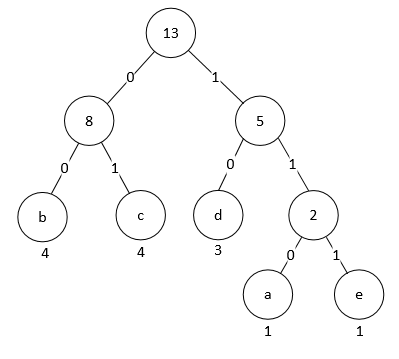The adjusted Huffman code table is :

character code
a 110
b 00
c 01
d 10
e 111

Note that the adjusted Huffman encoding does not affect the compression ratio: the length of each character encoding remains the same, and the encoding of the most frequently used characters is still the shortest. It is easy to see that encodings of the same length are incremental, and that the minimum value of an encoding of a certain length depends on the minimum value and number of encodings of the previous length. Let the minimum value of an encoding of length $i$ be $m_i$ and there are $n_i$; and let the minimum value of an encoding of length 0 be 0 and there are 0. Then it is easy to obtainThe “$\ll$” here means left shift. Thus, we only need to know the Huffman encoding length of each character to derive the Huffman encoding of all characters. For the above example, we just need to store the length sequence $<3, 2, 2, 2, 2, 3>$. When decompressing this sequence, we scan the sequence for the number of codes of each length, and find the minimum value of the codes of each length; codes of the same length are incremented sequentially, so we add one to the other to find the Huffman codes of all characters. In particular, a length of 0 means that the character does not appear in the compressed block and is excluded when constructing the Huffman code.

#### 3.2.2 Encoding length sequences

Length sequences are prone to having consecutive repetitive values; and since there may be many unused characters in the file, there may also be many zeros. Therefore DEFLATE encodes the length sequence as shown below:

code meaning
0-15 Indicates length 0 to 15
16 The first length is repeated 3 to 6 times. The next two digits indicate exactly how many times to repeat, similar to the length and distance codes in Section 3.2.
17 Length 0 is repeated 3 to 10 times. The next 3 digits indicate exactly how many times to repeat.
18 Length 0 is repeated 11 to 138 times. The next 7 digits indicate exactly how many times to repeat.

#### 3.2.3 Compressing Huffman Encoding Tables with Huffman Encoding

Representing the Huffman encoding table as a sequence of encoded lengths is already relatively compact, but the DEFLATE algorithm does not think it is enough. It decides to compress this encoded length sequence again using Huffman encoding. This step is a little bit more involved. In order to do this, we need to construct a Huffman encoding table for the encoding of the length sequence - 0 to 18 (however, this length sequence represents a Huffman encoding table, which means we are constructing a Huffman encoding table for a Huffman encoding table). The question arises, how to represent the Huffman encoding table of this Huffman encoding table? First, the DEFLATE algorithm defines a mystery array: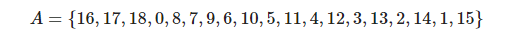Since it is possible that not all lengths will be used, we will first identify the first k codes in the array with a number. For example, if only length sequence codes 2, 3, 4, 16, 17 are used, then k = 16. The next k numbers will be $i$, with the first $i$ indicating the length of the Huffman code of Length Sequence Code $A[i]$, and 0 indicating that the corresponding code is not used. Since the Huffman encoding length of each character is known, the Huffman encoding of all characters can be deduced. This gives us the Huffman encoding table for the length sequence of encoding.

In the compressed data, we first store the Huffman encoding table of the encoded length sequence, followed by the Huffman encoding data of the encoded length sequence. This way, we store the Huffman encoding table of the compressed data in a small space in the compressed block.

## 4. The Gzip format

Finally, let’s briefly describe the gzip format.

The Gzip format consists of a header, a compression body, and a tail. The header contains basic information about the file, the time, the compression method, the operating system, etc. The tail contains the checksum and the original size. This information is not relevant to the compression algorithm, so we will not discuss it in depth here, but you can refer to RFC1952.

The compressed body consists of one or more blocks. Each block is of variable length, and has its own compression and encoding scheme. A block may start or end at any bit, without aligning the bytes. It is important to note that the distance indicated by the repeat marker may span the block.

All of the above are compressed using dynamic Huffman encoding. In fact, a block may also be compressed using static Huffman encoding or not. Blocks compressed with static Huffman encoding are compressed and decompressed using the algorithm’s predefined Huffman encoding, without storing the Huffman encoding table.

name length description
BFINAL 1 bit Identifies if this is the last block
BTYPE 2 bits Identifies the type. 00: uncompressed; 01: static Huffman encoding compression; 10: dynamic Huffman encoding compression; 11: reserved

### 4.1 Uncompressed blocks

The uncompressed block contains the following data (immediately after the header):

name length description
LEN 2 bits block data length
NLEN 2 bits LEN’s complement
DATA LEN bytes valid data

### 4.2 Compression blocks

The dynamic Huffman encoding compression block contains the following data (immediately after the header):

name length description
HLIT 5 bits Maximum length/character encoding minus 257. Since not all length encodings are used, the maximum length/character encoding is marked here (lengths share the same encoding space as normal characters).
HDIST 5 bits maximum value of distance encoding minus 1. Same principle as above.
HCLEN 4 bits k minus 4 in Section 3.2.3. Identifies that the first few codes in the mystery array are used.
The Huffman encoding table of the encoded length sequence (HCLEN + 4) * 3 bits k numbers as described in Section 3.2.3. Since there are only 19 length sequences encoded, 3 bits per digit is sufficient.
The Huffman encoding table for length/character encoding variable is used to represent the Huffman encoding table for length/character encoding based on the length sequence encoded in the Huffman encoding table above.
Distance-encoded Huffman table variable A sequence of lengths encoded by the above Huffman table, used to represent the distance-encoded Huffman table.
DATA variable valid compressed data
256 end-tags variable identify the end of the block

Static compression blocks are relatively simple, with the header followed by the valid compressed data, and finally a 256 end marker.

## 5. Summary

The DEFLATE algorithm is a very complex method to compress data as much as possible. Due to time and effort constraints, I have not read the zlib source code carefully, so this article is mainly based on RFC1951, and the implementation details are less discussed. If there are any errors or ambiguities, please feel free to correct them. For those who are interested in the implementation, please refer to the zlib source code.

BTW, there is a simple and efficient implementation of the LZ77 algorithm: ariya/FastLZ. FastLZ does not use Huffman encoding, only LZ77. It compresses text fast and well, but the compression ratio of binary data is not ideal. The FastLZ implementation is relatively simple and is recommended for reading.